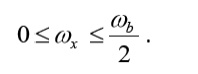Home | | Digital Communication | Nyquist Criterion for Zero-ISI

# Nyquist Criterion for Zero-ISI

Nyquist proposed a condition for pulses p(t) to have zero-ISI when transmitted through a channel with sufficient bandwidth to allow the spectrum of all the transmitted signal to pass. Nyquist proposed that a zero–ISI pulse p(t) must satisfy the condition

Nyquist Criterion for Zero-ISI

Nyquist proposed a condition for pulses p(t) to have zero–ISI when transmitted through a channel with sufficient bandwidth to allow the spectrum of all the transmitted signal to pass. Nyquist proposed that a zero–ISI pulse p(t) must satisfy the conditionA pulse that satisfies the above condition at multiples of the bit period Tb will result in zero– ISI if the whole spectrum of that signal is received. The reason for which these zero–ISI pulses (also called Nyquist–criterion pulses) cause no ISI is that each of these pulses at the sampling periods is either equal to 1 at the center of pulse and zero the points other pulses are centered.

In fact, there are many pulses that satisfy these conditions. For example, any square pulse that occurs in the time period –Tb to Tb or any part of it (it must be zero at –Tb and Tb) will satisfy the above condition.

Also, any triangular waveform („Δ‟ function) with a width that is less than 2Tb will also satisfy the condition. A sinc function that has zeros at t = Tb, 2Tb, 3Tb, … will also satisfy this condition. The problem with the sinc function is that it extends over a very long period of time resulting in a lot of processing to generate it. The square pulse required a lot of bandwidth to be transmitted. The triangular pulse is restricted in time but has relatively large bandwidth.

There is a set of pulses known as raised–cosine pulses that satisfy the Nyquist criterion and require slightly larger bandwidth than what a sinc pulse (which requires the minimum bandwidth ever) requires.

The spectrum of these pulses is given byWhere ω b is the frequency of bits in rad/s (ω b = 2 /Tb), and x is called the excess bandwidth and it defines how much bandwidth would be required above the minimum bandwidth that is required when using a sinc pulse. The excess bandwidth ω x for this type of pulses is restricted betweenSketching the spectrum of these pulses we getWe can easily verify that when ωx = 0, the above spectrum becomes a rect function, and therefore the pulse p(t) becomes the usual sinc function. For ωx = b/2, the spectrum is similar to a sinc function but decays (drops to zero) much faster than the sinc (it extends over 2 or 3 bit periods on each side). The expense for having a pulse that is short in time is that it requires a larger bandwidth than the sinc function (twice as much for ωxb/2). Sketch of the pulses and their spectrum for the two extreme cases of ω xb/2 and ωx = 0 are shown belowWe can define a factor r called the roll–off factor to beThe roll–off factor r specifies the ratio of extra bandwidth required for these pulses compared to the minimum bandwidth required by the sinc function.

Study Material, Lecturing Notes, Assignment, Reference, Wiki description explanation, brief detail
Digital Communication : Baseband Transmission : Nyquist Criterion for Zero-ISI |